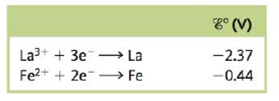# Consider a galvanic cell based on the following half-reactions: a. What is the expected cell potential with all components in their standard states? b. What is the oxidizing agent in the overall cell reaction? c. What substances make up the anode compartment? d. In the standard cell, in which direction do the electrons flow? e. How many electrons are transferred per unit of cell reaction? f. If this cell is set up at 25°C with [Fe 2+ ] = 2.00 × 10 −4 M and [La 3+ ] = 3.00 × 10 −3 M , what is the expected cell potential?### Chemistry: An Atoms First Approach

2nd Edition
Steven S. Zumdahl + 1 other
Publisher: Cengage Learning
ISBN: 9781305079243

#### Solutions

Chapter
Section### Chemistry: An Atoms First Approach

2nd Edition
Steven S. Zumdahl + 1 other
Publisher: Cengage Learning
ISBN: 9781305079243
Chapter 17, Problem 135CWP
Textbook Problem
1 views

## Consider a galvanic cell based on the following half-reactions:a. What is the expected cell potential with all components in their standard states?b. What is the oxidizing agent in the overall cell reaction?c. What substances make up the anode compartment?d. In the standard cell, in which direction do the electrons flow?e. How many electrons are transferred per unit of cell reaction?f. If this cell is set up at 25°C with [Fe2+] = 2.00 × 10−4 M and [La3+] = 3.00 × 10−3 M, what is the expected cell potential?

(a)

Interpretation Introduction

Interpretation:

Various questions based on the given galvanic cell have to be answered.

Concept introduction:

Galvanic cell is used for the conversion of chemical energy into electrical energy and it involves a spontaneous process, while the concentration cell is used for the conversion of electrical energy into chemical energy and it involves non-spontaneous process.

To determine: The cell potential with all the components in their standard states.

The value of cell potential with all the components in their standard states is 1.93V_ .

### Explanation of Solution

The reaction taking place at the cathode is,

Fe2++2eFeE°red=0.44V

The reaction taking place at the anode is,

LaLa3++3eE°=2.37V

Multiply reduction half-reaction with a coefficient of 3 and oxidation half-reaction with a coefficient of 2 and then add both the reactions as,

3Fe2++6e3Fe2La2La3++6e

The final equation is,

3Fe2++2La3Fe+2La3+

The value of E

(b)

Interpretation Introduction

Interpretation:

Various questions based on the given galvanic cell have to be answered.

Concept introduction:

Galvanic cell is used for the conversion of chemical energy into electrical energy and it involves a spontaneous process, while the concentration cell is used for the conversion of electrical energy into chemical energy and it involves non-spontaneous process.

To determine: The oxidizing agent in the overall cell reaction.

The oxidizing agent in the overall cell reaction is Fe2+ .

(c)

Interpretation Introduction

Interpretation:

Various questions based on the given galvanic cell have to be answered.

Concept introduction:

Galvanic cell is used for the conversion of chemical energy into electrical energy and it involves a spontaneous process, while the concentration cell is used for the conversion of electrical energy into chemical energy and it involves non-spontaneous process.

To determine: The substances that make up the anode compartment.

The substances that make up the anode compartment are Lanthanum and its electrolyte.

(d)

Interpretation Introduction

Interpretation:

Various questions based on the given galvanic cell have to be answered.

Concept introduction:

Galvanic cell is used for the conversion of chemical energy into electrical energy and it involves a spontaneous process, while the concentration cell is used for the conversion of electrical energy into chemical energy and it involves non-spontaneous process.

To determine: The direction of electrons in the standard cell.

The flow of electrons in the standard cell occurs from the anode to the cathode.

(e)

Interpretation Introduction

Interpretation:

Various questions based on the given galvanic cell have to be answered.

Concept introduction:

Galvanic cell is used for the conversion of chemical energy into electrical energy and it involves a spontaneous process, while the concentration cell is used for the conversion of electrical energy into chemical energy and it involves non-spontaneous process.

To determine: The direction of electrons in the standard cell.

The flow of electrons in the standard cell occurs from the anode to the cathode.

(f)

Interpretation Introduction

Interpretation:

Various questions based on the given galvanic cell have to be answered.

Concept introduction:

Galvanic cell is used for the conversion of chemical energy into electrical energy and it involves a spontaneous process, while the concentration cell is used for the conversion of electrical energy into chemical energy and it involves non-spontaneous process.

To determine: The expected cell potential for the given concentration of ions.

The expected cell potential for the given concentration of ions is 1.9V_ .

### Still sussing out bartleby?

Check out a sample textbook solution.

See a sample solution

#### The Solution to Your Study Problems

Bartleby provides explanations to thousands of textbook problems written by our experts, many with advanced degrees!

Get Started

Find more solutions based on key concepts
Blood carries nutrients absorbed from food a. from the intestine to the liver. b. from the lungs to the extremi...

Nutrition: Concepts and Controversies - Standalone book (MindTap Course List)

What are genomes?

Human Heredity: Principles and Issues (MindTap Course List)

An inclined plane of angle = 20.0 has a spring of force constant k = 500 N/m fastened securely at the bottom s...

Physics for Scientists and Engineers, Technology Update (No access codes included)

What is a coastal cell? Where does sand in a coastal cell come from? Where does it go?

Oceanography: An Invitation To Marine Science, Loose-leaf Versin